true

Ram Mohan A.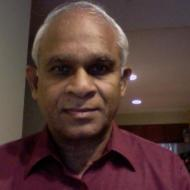Featured

# Ram Mohan A.

## TEACHING IS MY PASSION-I STILL STRIVE TO BETTER MY...

130 Students taught

Referral Discount: Get ₹ 500 off when you make a payment to start classes. Get started by Booking a Demo.

Details verified of Ram Mohan A.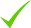IdentityEducation

Know how UrbanPro verifies Tutor details

Identity is verified based on matching the details uploaded by the Tutor with government databases.

## Overview

I am a Post Graduate in Nuclear Physics. Aged 61 yrs with a teaching experience of about 25 yrs.

Can teach MATHS, PHYSICS, CHEMISTRY FOR 8th - 10th Standards (CAN TEACH PHYSICS UP TO 12th Standard)

I have a special passion to TEACH VEDIC ARITHMETIC

Intro Video

• Class 9 Tuition , Class 10 Tuition +3

## Languages Spoken

Telugu Mother Tongue (Native)

English Proficient

## Education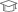GOVERNMENT DEGREE COLLEGE, RAZOLE, E.G.Dst, ANDHRA PRADESH 1973

Bachelor of Science (B.Sc.)NUCLEAR RESEARCH LANORATORIES, ANDHRA UNIVERSITY 1975

MSc IN NUCLEAR PHYSICS

## Verified Info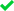ID VerifiedPhone VerifiedEmail Verified

Report this Profile

Is this listing inaccurate or duplicate? Any other problem?

Type the letters as shown below *

Please enter the letters as show below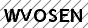Class 10 Tuition

Class LocationOnline Classes (Video Call via UrbanPro LIVE)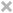Student's HomeTutor's Home

Years of Experience in Class 10 Tuition

20

Board

CBSE, State, International Baccalaureate, ICSE

IB Subjects taught

Physics, Chemistry, Mathematics

CBSE Subjects taught

Mathematics

ICSE Subjects taught

Chemistry, Mathematics, Physics

Taught in School or College

Yes

State Syllabus Subjects taught

Mathematics

Teaching Experience in detail in Class 10 Tuition

Teaching is my Passion. I have taught Maths, Physics and Chemistry to several students during 20 years of my teaching. Many of my students who initially were scarred of Maths, have developed interest on the subject and opted MPC group in their Intermediate and are now earning Dollars as Software Engineers in USA,CANADA,UK and Australia.

## Reviews (19)

this is test message this is test message this is test message this is test message this is test message this is test message this is test message

4.9 out of 5 19 reviews

RAM MOHAN A. https://s3-ap-southeast-1.amazonaws.com/tv-prod/member/photo/226856-small.jpg Serlingampally
4.90519
RAM MOHAN A.
M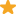"A very good teacher and a knowledgeable one also. I have seen improvement in my grades before and after joining the classes. During my exam times the revision given by teacher was very helpful. "

RAM MOHAN A.
M"Very Good classes. Great explanation of topic. Always helped with step-by-step explanation. Very Helpful. "

RAM MOHAN A.
VI loved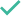Teacher's KnowledgeTeaching MethodClass ContentAudio/Video Quality

RAM MOHAN A.
SI lovedClass ContentTeaching Method

Have you attended any class with Ram Mohan? Write a Review

## FAQs

1. Which school boards of Class 10 do you teach for?

CBSE, State, International Baccalaureate and others

2. Do you have any prior teaching experience?

Yes

3. Which classes do you teach?

I teach Class 10 Tuition, Class 7 Tuition, Class 8 Tuition and Class 9 Tuition Classes.

4. Do you provide a demo class?

Yes, I provide a free demo class.

5. How many years of experience do you have?

I have been teaching for 20 years.

## Answers by Ram Mohan (38)

If 'r' stands for the percentage rate of Sales Tax say then (100+r) will be the Sale Price. While its cost price is 100 and 'r' will be the Sales Tax '. Therefore, if 'SP stands for actual Sale Price and 'ST' stands for actual Sales tax then we have:- (100+r) : r :: SP : ST OR (100+r)/r = SP / ST... ...more
If 'r' stands for the percentage rate of Sales Tax say then (100+r) will be the Sale Price. While its cost price is 100 and 'r' will be the Sales Tax '. Therefore, if 'SP stands for actual Sale Price and 'ST' stands for actual Sales tax then we have:- (100+r) : r :: SP : ST OR (100+r)/r = SP / ST From this formula , given the values of any two of 'r' ; 'SP' ; 'ST' the value of the third can be found. In the given Sum, r = 10, SP = 17600 ST =? from the above formula, we can write ST =r * SP /(100+r) = 10 * 17600 /(100+10) = 10 * 17600/110 = Rs.1600. Therefore, the Sales Tax on the Refrigerator is Rs.1600.
Dislike Bookmark

If l, b, h, S and V are length, breadth, height, surface area and volume respectively of a cuboid, Then We have S = 2( lb + bh + lh ) and V = lbh Let us take the relation S = 2(lb +bh + lh). Now dividing both sides with V * S, we get 1/V = 2 ( lb + bh = lh )/ (V * S) = 2 ( lb + bh +lh )/ (lbh *... ...more
If l, b, h, S and V are length, breadth, height, surface area and volume respectively of a cuboid, Then We have S=2( lb + bh + lh ) and V=lbh Let us take the relation S=2(lb +bh + lh). Now dividing both sides with V * S, we get 1/V = 2 ( lb + bh=lh )/ (V * S) = 2 ( lb + bh +lh )/ (lbh * S) ...... ( after Substituting lbh for V) ==> 1/V = 2 [(lb/lbh )+( bh/lbh ) + (lh/lbh )] / S==> 1/V = 2/S(1/l + 1/b + 1/h) proved.
Dislike Bookmark

If l, b, h, S and V are length, breadth, height, surface area and volume respectively of a cuboid, Then, V = lbh Let us take the relation S = 2 (lb +bh + lh). Now dividing both sides with V * S, we get:- 1/V = 2 ( lb + bh = lh )/ (V * S) = 2 ( lb + bh = lh )/ (lbh * S) ...... ( Substituting lbh for... ...more
If l, b, h, S and V are length, breadth, height, surface area and volume respectively of a cuboid, Then, V=lbh Let us take the relation S=2 (lb +bh + lh). Now dividing both sides with V * S, we get:- 1/V = 2 ( lb + bh=lh )/ (V * S) = 2 ( lb + bh=lh )/ (lbh * S) ...... ( Substituting lbh for V) ==> 1/V = =2 [(lb/lbh )+( bh/lbh ) + (lh/ibh )] / S==> 1/V = 2/S(1/l + 1/b + 1/h) proved.
Dislike Bookmark

Given that A:B = 4:5, B:C = 6:7 and C:D = 14:15. Therefore, A/B =4/5; B/A = 6/7; C/D = 14/15 We can write A/D as A/D = A/B * B/C *C/D = 4/5 * 6/7 * 14/15 = 16/25 Thus, A : D = 16 : 25.
Dislike Bookmark

Find constant A such that log base 3 x=A log base 5 x for all x > 0?

We know that, log 'x' to base 'y' = log 'x' to base ' z ' * log'z' to base' y' ............. (1) and log 'p' to base 'q' = 1/ log 'q' to base 'x' ............(2) In the present context, It is given that log 'x' to base '3' = A log 'x'to base '5' by using formula (2) above, we can rewrite this equation... ...more
We know that, log 'x' to base 'y' = log 'x' to base ' z ' * log'z' to base' y' ............. (1) and log 'p' to base 'q' = 1/ log 'q' to base 'x' ............(2) In the present context, It is given that log 'x' to base '3' = A log 'x'to base '5' by using formula (2) above, we can rewrite this equation as:- log 'x' to base '3' = A * 1/ log '5' to base 'x' ==> log '5' to base 'x' * log 'x' to base '3' = A==> log '5' to base '3' = A ( [By using the Formula (1) above, (log'5' to base 'x' * log 'x' to base '3') becomes (" log '5' to base '3'). Therefore, A = log 5 to base 3.
Dislike Bookmark
Class 10 Tuition 5.0

Class LocationOnline Classes (Video Call via UrbanPro LIVE)Student's HomeTutor's Home

Years of Experience in Class 10 Tuition

20

Board

CBSE, State, International Baccalaureate, ICSE

IB Subjects taught

Physics, Chemistry, Mathematics

CBSE Subjects taught

Mathematics

ICSE Subjects taught

Chemistry, Mathematics, Physics

Taught in School or College

Yes

State Syllabus Subjects taught

Mathematics

Teaching Experience in detail in Class 10 Tuition

Teaching is my Passion. I have taught Maths, Physics and Chemistry to several students during 20 years of my teaching. Many of my students who initially were scarred of Maths, have developed interest on the subject and opted MPC group in their Intermediate and are now earning Dollars as Software Engineers in USA,CANADA,UK and Australia.

Class 9 Tuition 5.0

Class LocationOnline Classes (Video Call via UrbanPro LIVE)Student's HomeTutor's Home

Years of Experience in Class 9 Tuition

20

Board

CBSE, State, International Baccalaureate, ICSE

IB Subjects taught

Physics, Chemistry, Mathematics

CBSE Subjects taught

Mathematics

ICSE Subjects taught

Chemistry, Mathematics, Physics

Taught in School or College

Yes

State Syllabus Subjects taught

Mathematics

Teaching Experience in detail in Class 9 Tuition

Teaching is my Passion. I have taught Maths, Physics and Chemistry to several students during 20 years of my teaching. Many of my students who initially were scarred of Maths, have developed interest on the subject and opted MPC group in their Intermediate and are now earning Dollars as Software Engineers in USA,CANADA,UK and Australia.

Class 8 Tuition 5.0

Class LocationOnline Classes (Video Call via UrbanPro LIVE)Student's HomeTutor's Home

Years of Experience in Class 8 Tuition

20

Board

ICSE, State, CBSE

CBSE Subjects taught

Mathematics, Science

ICSE Subjects taught

Mathematics, Chemistry, Physics

Taught in School or College

Yes

State Syllabus Subjects taught

Mathematics, Science

Teaching Experience in detail in Class 8 Tuition

I have taught in coaching centers and Tutorial colleges,besides running my own Tution batches for about 25 years, since 1983.

Class 7 Tuition 5.0

Class LocationOnline Classes (Video Call via UrbanPro LIVE)Student's HomeTutor's Home

Years of Experience in Class 7 Tuition

20

Board

ICSE, State, CBSE

CBSE Subjects taught

Mathematics, Science

ICSE Subjects taught

Mathematics, Chemistry, Physics

Taught in School or College

Yes

State Syllabus Subjects taught

Mathematics, Science

Teaching Experience in detail in Class 7 Tuition

I have taught in coaching centers and Tutorial colleges,besides running my own Tution batches for about 25 years, since 1983.

## Answers by Ram Mohan A. (38)

If 'r' stands for the percentage rate of Sales Tax say then (100+r) will be the Sale Price. While its cost price is 100 and 'r' will be the Sales Tax '. Therefore, if 'SP stands for actual Sale Price and 'ST' stands for actual Sales tax then we have:- (100+r) : r :: SP : ST OR (100+r)/r = SP / ST... ...more
If 'r' stands for the percentage rate of Sales Tax say then (100+r) will be the Sale Price. While its cost price is 100 and 'r' will be the Sales Tax '. Therefore, if 'SP stands for actual Sale Price and 'ST' stands for actual Sales tax then we have:- (100+r) : r :: SP : ST OR (100+r)/r = SP / ST From this formula , given the values of any two of 'r' ; 'SP' ; 'ST' the value of the third can be found. In the given Sum, r = 10, SP = 17600 ST =? from the above formula, we can write ST =r * SP /(100+r) = 10 * 17600 /(100+10) = 10 * 17600/110 = Rs.1600. Therefore, the Sales Tax on the Refrigerator is Rs.1600.
Dislike Bookmark

If l, b, h, S and V are length, breadth, height, surface area and volume respectively of a cuboid, Then We have S = 2( lb + bh + lh ) and V = lbh Let us take the relation S = 2(lb +bh + lh). Now dividing both sides with V * S, we get 1/V = 2 ( lb + bh = lh )/ (V * S) = 2 ( lb + bh +lh )/ (lbh *... ...more
If l, b, h, S and V are length, breadth, height, surface area and volume respectively of a cuboid, Then We have S=2( lb + bh + lh ) and V=lbh Let us take the relation S=2(lb +bh + lh). Now dividing both sides with V * S, we get 1/V = 2 ( lb + bh=lh )/ (V * S) = 2 ( lb + bh +lh )/ (lbh * S) ...... ( after Substituting lbh for V) ==> 1/V = 2 [(lb/lbh )+( bh/lbh ) + (lh/lbh )] / S==> 1/V = 2/S(1/l + 1/b + 1/h) proved.
Dislike Bookmark

If l, b, h, S and V are length, breadth, height, surface area and volume respectively of a cuboid, Then, V = lbh Let us take the relation S = 2 (lb +bh + lh). Now dividing both sides with V * S, we get:- 1/V = 2 ( lb + bh = lh )/ (V * S) = 2 ( lb + bh = lh )/ (lbh * S) ...... ( Substituting lbh for... ...more
If l, b, h, S and V are length, breadth, height, surface area and volume respectively of a cuboid, Then, V=lbh Let us take the relation S=2 (lb +bh + lh). Now dividing both sides with V * S, we get:- 1/V = 2 ( lb + bh=lh )/ (V * S) = 2 ( lb + bh=lh )/ (lbh * S) ...... ( Substituting lbh for V) ==> 1/V = =2 [(lb/lbh )+( bh/lbh ) + (lh/ibh )] / S==> 1/V = 2/S(1/l + 1/b + 1/h) proved.
Dislike Bookmark

Given that A:B = 4:5, B:C = 6:7 and C:D = 14:15. Therefore, A/B =4/5; B/A = 6/7; C/D = 14/15 We can write A/D as A/D = A/B * B/C *C/D = 4/5 * 6/7 * 14/15 = 16/25 Thus, A : D = 16 : 25.
Dislike Bookmark

Find constant A such that log base 3 x=A log base 5 x for all x > 0?

We know that, log 'x' to base 'y' = log 'x' to base ' z ' * log'z' to base' y' ............. (1) and log 'p' to base 'q' = 1/ log 'q' to base 'x' ............(2) In the present context, It is given that log 'x' to base '3' = A log 'x'to base '5' by using formula (2) above, we can rewrite this equation... ...more
We know that, log 'x' to base 'y' = log 'x' to base ' z ' * log'z' to base' y' ............. (1) and log 'p' to base 'q' = 1/ log 'q' to base 'x' ............(2) In the present context, It is given that log 'x' to base '3' = A log 'x'to base '5' by using formula (2) above, we can rewrite this equation as:- log 'x' to base '3' = A * 1/ log '5' to base 'x' ==> log '5' to base 'x' * log 'x' to base '3' = A==> log '5' to base '3' = A ( [By using the Formula (1) above, (log'5' to base 'x' * log 'x' to base '3') becomes (" log '5' to base '3'). Therefore, A = log 5 to base 3.
Dislike Bookmark

Ram Mohan A. describes himself as TEACHING IS MY PASSION-I STILL STRIVE TO BETTER MY TEACHING ABILITIES. He conducts classes in Class 10 Tuition, Class 7 Tuition and Class 8 Tuition. Ram Mohan is located in Serlingampally, Hyderabad. Ram Mohan takes Online Classes- via online medium. He has 20 years of teaching experience . Ram Mohan has completed Bachelor of Science (B.Sc.) from GOVERNMENT DEGREE COLLEGE, RAZOLE ,E.G.Dst, ANDHRA PRADESH in 1973 and MSc IN NUCLEAR PHYSICS from NUCLEAR RESEARCH LANORATORIES, ANDHRA UNIVERSITY in 1975. He is well versed in English and Telugu. Ram Mohan has got 19 reviews till now with 47% positive feedback.

•• Want to learn from Ram Mohan?

• Book a Demo
X

Recommended Profiles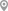Serlingampally, HyderabadTrimulgherry, HyderabadSerlingampally, HyderabadBowenpally, HyderabadBalamrai, HyderabadAPHB Colony, Hyderabad

X

Let us shortlist and give the best tutors and institutes.

or

Send Enquiry to Ram Mohan

Let Ram Mohan know you are interested in their class